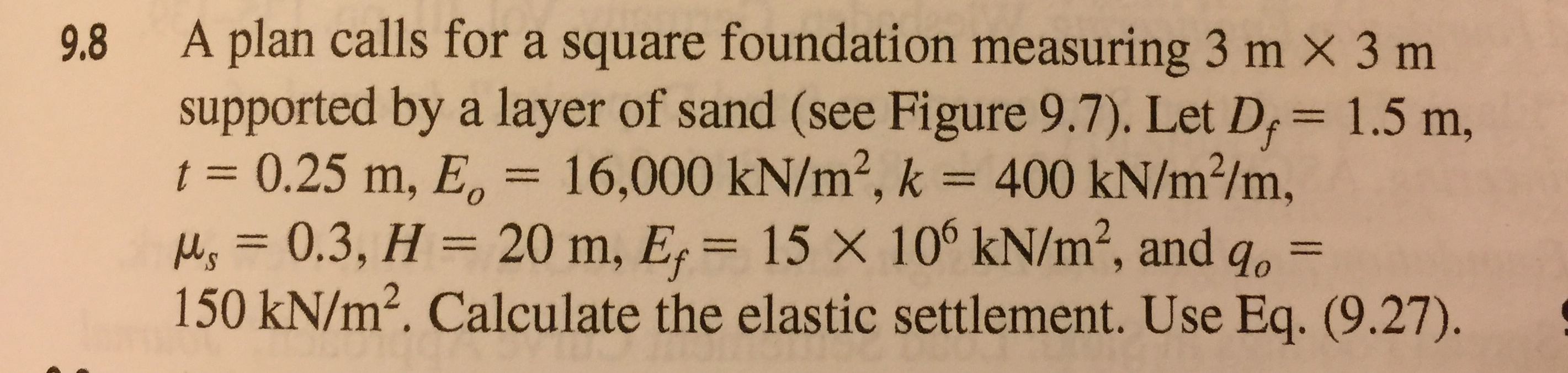9.8 A plan calls for a square foundation measuring 3 m x 3 msupported by a layer of sand (see Figure 9.7). Let D, 1.5 m,t 0.25 m, E 16,000 kN/m2, k 400 kN/m2/m,, 0.3, H 20 m, E 15 X 10° kN/m2, and go-150 kN/m2. Calculate the elastic settlement. Use Eq. (9.27).

Question

subject : Geotechnical Design

Book : PRINCIPLE OF FOUNDATION ENGINEERINGhelp_outlineImage Transcriptionclose9.8 A plan calls for a square foundation measuring 3 m x 3 m supported by a layer of sand (see Figure 9.7). Let D, 1.5 m, t 0.25 m, E 16,000 kN/m2, k 400 kN/m2/m, , 0.3, H 20 m, E 15 X 10° kN/m2, and go- 150 kN/m2. Calculate the elastic settlement. Use Eq. (9.27). fullscreen
Step 1

We have for elastic settlement the following equation:

Step 2

Following diagram explains the given conditions:

Step 3

Let’s find out the value of Be...

Want to see the full answer?

See Solution

Want to see this answer and more?

Our solutions are written by experts, many with advanced degrees, and available 24/7

See Solution
Tagged in

Civil Engineering